Using the Error Function (Erf) Instead

These areas can also be found by using the "Error Function" or "Erf", which is the area starting at z=0 when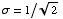.
(Internally this is what Mathematica does, treating Erf[x] as a built-in like Sin[x].)
So the integral of the standard normal distribution from 0 to z is also given by 0.5 Erf[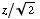].
For example, here is the area under the normal curve from z=0 to z=1, calculated to 20 places, two ways.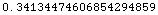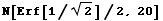Or, going the other way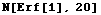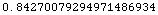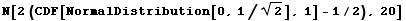Mathematica also defines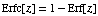(Erf complement) which gives us another way to define f[z] = areaLeftOfZNormal[z].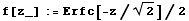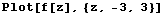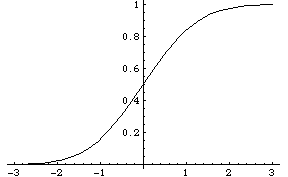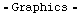Inverting this gives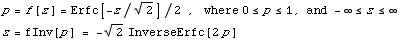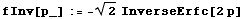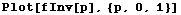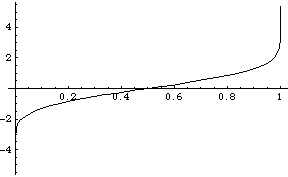So the total probability under the standard (μ=0,σ=1) Normal curve, for z ≤ 1 is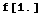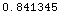And the value of z for which the probability is 0.841345 is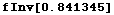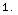Created by Mathematica  (October 2, 2003)